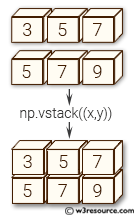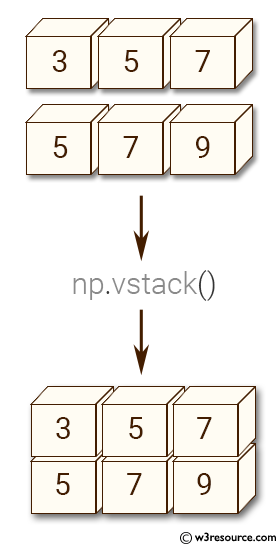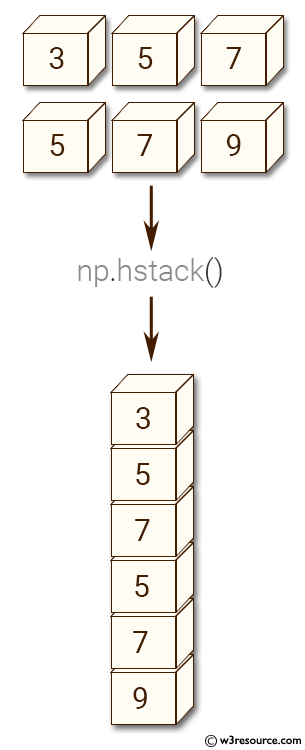# NumPy Array manipulation: vstack() function

## numpy.vstack() function

The vstack() function is used to stack arrays in sequence vertically (row wise).
This is equivalent to concatenation along the first axis after 1-D arrays of shape (N,) have been reshaped to (1,N).
This function makes most sense for arrays with up to 3 dimensions. For instance, for pixel-data with a height (first axis), width (second axis), and r/g/b channels (third axis). T

Syntax:

`numpy.vstack(tup)`Version: 1.15.0

Parameter:

Name Description Required /
Optional
tup The arrays must have the same shape along all but the first axis. 1-D arrays must have the same length. Required

Return value:

stacked : ndarray The array formed by stacking the given arrays.

Example-1: numpy.vstack() function

``````>>> import numpy as np
>>> x = np.array([3, 5, 7])
>>> y = np.array([5, 7, 9])
>>> np.vstack((x,y))
array([[3, 5, 7],
[5, 7, 9]])
``````

Pictorial Presentation:Example-2: numpy.vstack() function

``````>>> import numpy as np
>>> x = np.array([, , ])
>>> y = np.array([, , ])
>>> np.vstack((x,y))
array([,
,
,
,
,
])
``````

Pictorial Presentation:Python - NumPy Code Editor:

Previous: hstack()
Next: block()

﻿